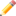## FANDOM

410 PagesThis article is a stub. You can help Programmer's Wiki by expanding it.Remember to remove this when the stub is fixed.

A do-while loop is a loop which executes at least once and will only terminate when the Boolean expression (or "condition") returns false. The do-while loop is not as popular as the while loop because any references inside it which depend on the condition being true must be usable before the condition is checked. The do-while loop is often used when some preparation is needed before iteration.

## Curly bracket programming languagesEdit

```int x = 0;
int y = 10;

do {
x++;
} while( x < y );```

## PascalEdit

```repeat
x := x + 1;
until (x < y);```

## Visual BasicEdit

Visual Basic's loops work similar to other languages:

```Dim x As Integer = 0
Dim y As Integer = 10

Do
x += 1
Loop While x < y```

However, the while statement can appear on the "DO" line, where the condition is executed first:

```Do While x < y
x += 1
Loop```

It may also be replaced with Until, which repeats the loop as long as the condition is false:

```Do
x += 1
Loop Until x >= y```

## PythonEdit

Python does not have a Do-while loop so if you want to use one you have to be a bit tricky.

```while True:
do_something()
if condition():
break```
Control structures
If statement - Switch statement - While loop - For loop - Foreach loop - Do while loop